### Civil Engineering Pack for Android Tablet##### Summary:
574 Calculators & Converters related to Civil, Beams, Columns, Piling, Concrete, Survey, Soil & Earthwork, Structural Engineering, Bridges, Highway & Road, Hydraulics and Timber.
1.0
Android
##### Category:
Calculator,Personal Productivity
\$15.99
##### Requiements:
Works with all Android Tablet
##### Overview

Civil Engineering Pack contains 574 Calculators and Converters, that can quickly and easily calculate and convert different Civil Engineering parameters. Automatic & Accurate Calculations and Conversions with every Unit and Value Changes. Available in Imperial and Metric Units. Most Comprehensive Civil Engineering and Construction Calculator.

Civil Engineering Pack contains the following 12 modules:
• Beam Calculator
• Column Calculator
• Piles and Piling Calculator
• Concrete Calculator
• Engineering Survey Calculator
• Soil and Earthwork Calculator
• Structural Engineering Calculator
• Bridge Calculator
• Hydraulics and Waterworks Calculator
• Timber Engineering Calculator
• Unit Converter

Civil Engineering Pack - SI Units (Metric System)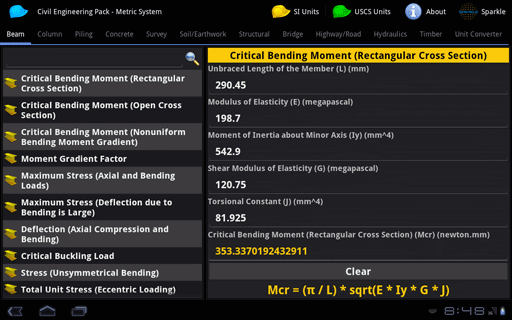Civil Engineering Pack - USCS Units (Imperial System)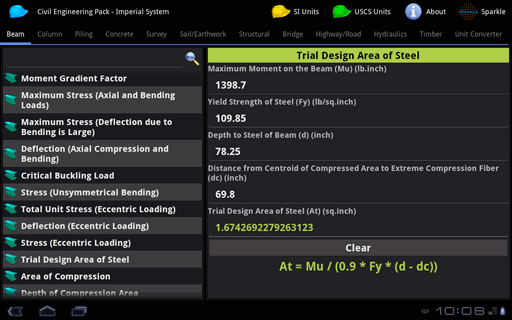Available in Metric and Imperial Units

Beam Calculator contains 34 Calculators that can quickly and easily calculate different Beam parameters.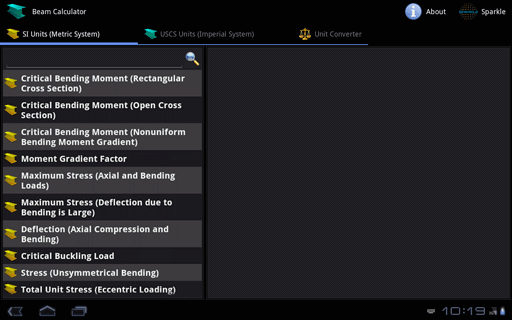Column Calculator contains 35 Calculators that can quickly and easily calculate different Column parameters.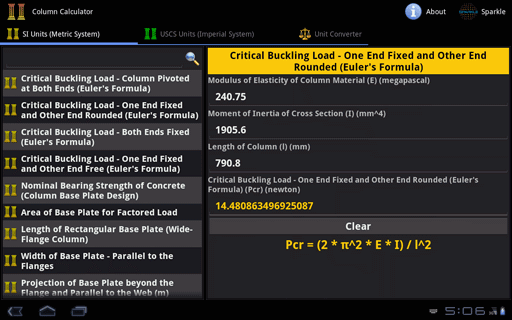Piles & Piling Calculator contains 22 Calculators that can quickly and easily calculate different Piles and Piling parameters.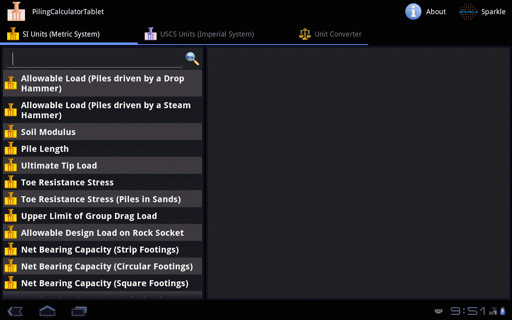Concrete Calculator contains 56 Calculators that can quickly and easily calculate different Concrete parameters.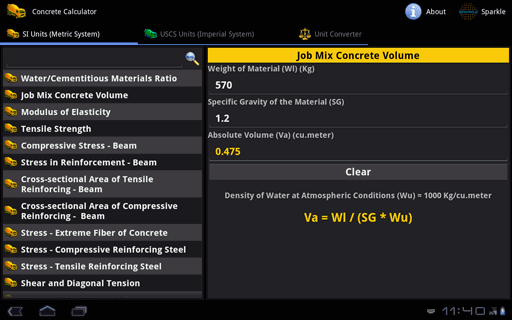Engineering Survey Calculator contains 33 Calculators that can quickly and easily calculate different Surveying parameters.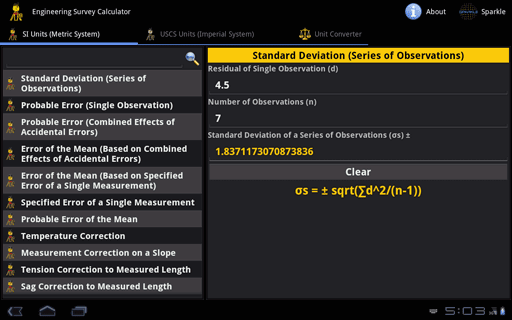Soil & Earthwork Calculator contains 60 Calculators that can quickly and easily calculate different Soil and Earthwork parameters.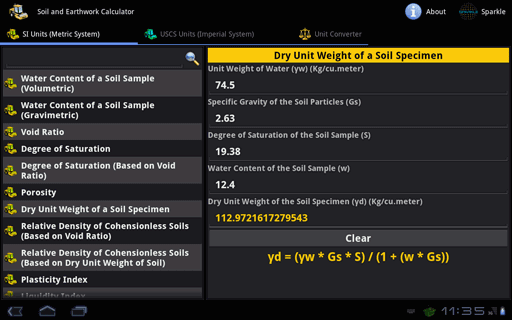Structural Engineering Calculator contains 58 Calculators that can quickly and easily calculate different Structural Engineering parameters.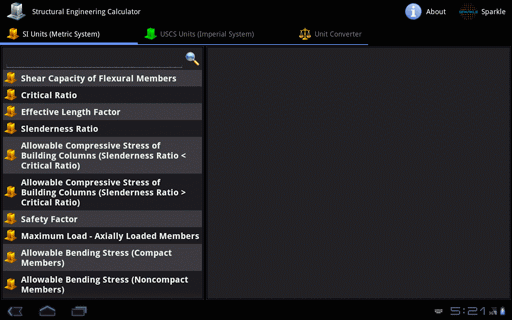Bridge Calculator contains 58 Calculators that can quickly and easily calculate different Bridge and Suspension Cable parameters.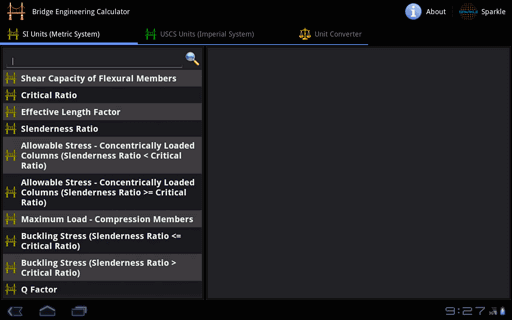Highway & Road Calculator contains 37 Calculators that can quickly and easily calculate different Highway and Road parameters.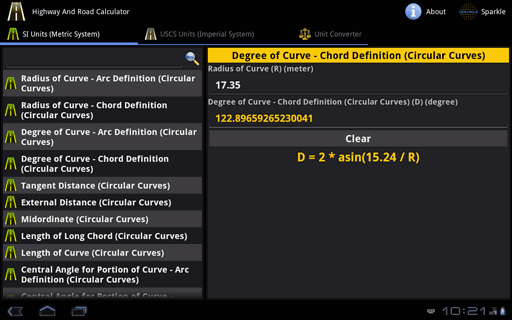Hydraulics & Waterworks Calculator contains 94 Calculators that can quickly and easily calculate different Hydraulics and Waterworks parameters.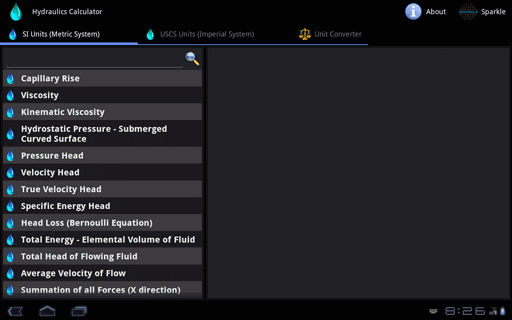Timber Engineering Calculator contains 55 Calculators that can quickly and easily calculate different Timber Engineering parameters.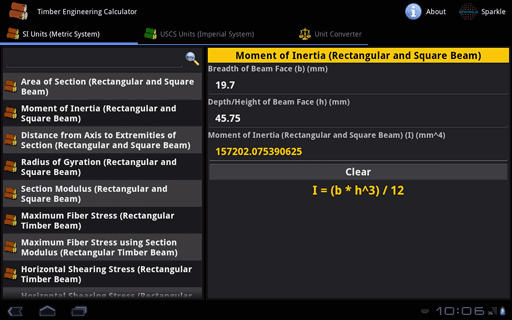Unit Converter contains following 32 Converters:

• Acceleration
• Angle
• Area
• Density
• Energy/Work
• Flow Rate (Mass)
• Flow Rate (Volume)
• Fluid
• Force
• Frequency
• Hardness
• Length
• Mass
• Metric Weight
• Metrology
• Moment of Force
• Moment of Inertia
• Prefixes
• Pressure
• Specific Heat Capacity
• Specific Volume
• Temperature
• Thermal Conductivity
• Thermal Expansion
• Time
• Torque
• Velocity
• Viscosity (Dynamic)
• Viscosity (Oil & Water)
• Viscosity (Kinematic)
• Volume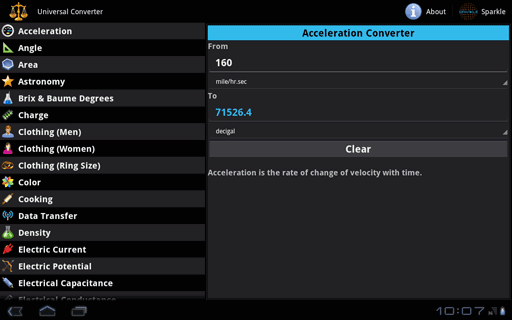Key Features:
• All Calculators are available both in SI Units (Metric System) and USCS Units (Imperial System).
• Complete coverage of calculators and converters in Civil Engineering and Construction Parameters.
• Automatic Calculation & Conversion of the Output with respect to changes in the Input/Options/Units.
• Formulas are provided for each calculator.
• Values of Higher Order can also be calculated.
• Extremely Accurate Calculations and Conversions.
• Professionally and Newly designed user-interface that speeds up Data Entry, Easy Viewing and Calculation Speed.
Most Comprehensive Civil and Construction Calculator Pack

•  Traze - reach ur friends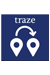Share location with friends & view theirs. Navigate & make calls, all in one go. Traze enables two-way communication. Its never been easier to reach someone. More...Civil Engineering Pack for Android Tablet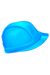574 Calculators & Converters related to Civil, Beams, Columns, Piling, Concrete, Survey, Soil & Earthwork, Structural Engineering, Bridges, Highway & Road, Hydraulics and Timber. More...